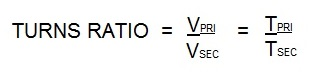What is the difference between 47 turns ratio and 45 turns ratio?

The answer is two more coil turns in the primary of the transformer with the 47 vs the 45. This is not a lot of difference. Let’s discuss what turns ratio is. Turns ratio refers to the number of times the copper primary is coiled around the steel core in an AC transformer. For more information go to the article:

“WHAT IS TRANSFORMER TURNS RATIO?”

In it the following formula is given:Vpri = Voltage on the primary                     Tpri = Turns in the primary coil

Vsec= Voltage on the secondary                Tsec = Turns in the secondary coil

An AC secondary coil has one turn. So for easy math using 220 V input. The above formula results in:

The 47 turns would produce 4.7 V.
The 45 turns would produce 4.9 V

This is the voltage in the secondary circuit of the transformer. By changing the turns ratio we were able to change the voltage output of the transformer.  Some transformers have tap switches built in.  These tap leads connect to various points in the primary coil. This allows you to change the turns ratio of a transformer which gives you a multitude of voltages from one transformer.

See Article: WHAT IS THE LOWEST TRANSFORMER TAP?

Reference: RWMA Manual Chapter 19

Have a Question?

Do you have a question that is not covered in our knowledgebase? Do you have questions regarding the above article? Click here to ask the professor.